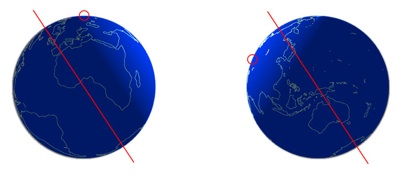Science Kids: Providing answers to some of those perplexing questions about Science and Nature.

WHY IS THE SKY BLUE?

Gas molecules in the atmosphere scatter, in all directions, the short wavelength light that appears blue to us. Longer wavelength light is largely unaffected as it passes through the atmosphere. As a result, when you look at the sky, you see blue everywhere. Read on for a more detailed explanation.

 Fact 1. Light travels in waves. The light's wavelength determines its color. Short wavelength light, for example, appears blue, and long wavelength light appears red.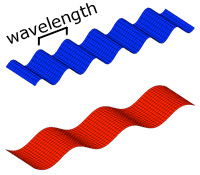Fact 2. When light strikes particles that are larger than its wavelength, the light's path may be altered. When light strikes particles that are smaller than its wavelength, the light continues to travel unaffected.Fact 3. The atmosphere contains many particles and gases, mainly nitrogen and oxygen.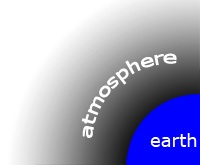Sunlight is composed of light of many different wavelengths. Longer wavelength light appears red, orange, and yellow, while shorter wavelength light appears blue, indigo and violet. The gas molecules in the atmosphere scatter, in all directions, shorter wavelength light (e.g., blue). The longer wavelegnth light (e.g., red) is largely unaffected by the atmosphere. As a result, when you look at the sky, you see the blue portion of the sun's light being scattered by the atmosphere. If you were to look at the sky while standing on the moon, you would see a very bright star surrounded by complete darkness. This is because the moon has no atmosphere and so sunlight is not scattered.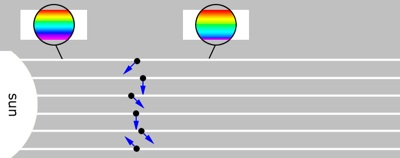You might wonder why the sky is not the color of the even shorter wavelength violet. The primary reason for this is that our eyes are better at detecting blue light than they are at detecting violet light.

HOW DO AIRPLANES FLY?

When an airplane is in flight, there is a downward force (gravity) and an upward force (lift) acting on the airplane. As an airplane moves through the air, the shape of the wings causes there to be less air pressure pushing down on the wings than pushing up on the wings. This difference in pressure yields the upward lift. If the downward gravitational force is less than the upward lift, then the airplane stays in the air. Read on for a more detailed explanation.

 Fact 1. As an airplane's propellers spin, they give the airplane a forward force (thrust). As the airplane moves forward there is a backward force (drag) caused by the resistance of the air.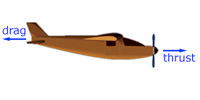Fact 2. An airplane is attracted downward due to gravity. This force increases with the mass (weight) of the object.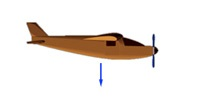Fact 3. As an airplane moves forward, the shape of its wing creates lower pressure above the wing than below, yielding a net upward force known as lift.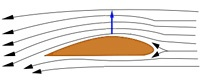As an airplane speeds along the runway, the airplane's wings disrupt the flow of the air. This disruption causes the air above the wings to move faster than below the wings. As a result, the air pressure above the wing is less than below the wing. As the airplane speeds up, this pressure difference increases until the lift is stronger than the gravitational force, and the airplane takes off. In order to remain in flight, the thrust must be greater than the drag, and lift must be greater than the force of gravity.

There is still some debate as to the exact cause of the difference in the air's speed around the wings. The basic concept described here, however, seems to explain why an airplane can fly.

HOW ARE RAINBOWS FORMED?

Sunlight is composed of light of varying wavelengths. Short wavelength light appears blue, violet and indigo, and long wavelength light appears red, orange and yellow. When sunlight enters a raindrop in the air, the light splits into a multitude of colors. This light then reflects off the back of the raindrop and re-emerges in the direction in which the light first entered. The light emerging from many raindrops creates a rainbow. Read on for a more detailed explanation.

 Fact 1. Light travels in waves. The light's wavelength determines its perceived color. Short wavelength light, for example, appears blue, and long wavelength light appears red.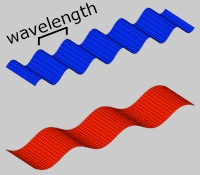Fact 2. Sunlight is composed of light of many wavelengths. In the range that we can see, this includes the colors of the rainbow.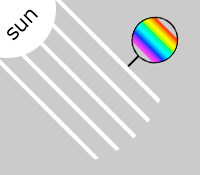Fact 3. When light enters water it bends (refracts). The amount of bending depends on the wavelength of light. As a result, the light splits into its component colors.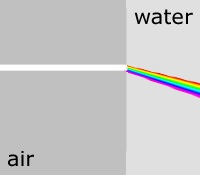When a ray of sunlight enters a raindrop it bends (refracts). The light then strikes the back of the raindrop, where some of the light passes through and some is reflected. As the light exits the raindrop, it is refracted again. The angle at which the light emerges depends on the wavelength of light. This path is illustrated in the small box below, where only the bending of two wavelengths (blue and red) are shown.

Consider now the diagram on the left. The sun is behind you (white rays) and there is rain in front of you (black dots). As the sunlight enters each raindrop, the light is refracted and reflected as described above. Because the sun is so far away, the rays of sunlight are nearly parallel to one another. As a result, the angle between the red line and each ray of sunlight striking a raindrop on that line will be the same. So, the light that reaches your eye along this ray will be of the same wavelength (color). The same is true for the yellow, blue and intermediate lines corresponding to each color of the rainbow.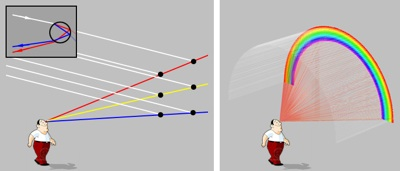Consider now the diagram on the right which explains why the colors of a rainbow form an arc. The angle between the incoming rays of sunlight (white) and all of the red lines, forming a circular cone, have the same angle. As a result, the light that reaches your eye along these lines have the same wavelength (color). The same is true for each band of the rainbow.

The reason that rainbows are somewhat rare is that you will only see them when there is rain in front of you and somewhat in the distance, and the sun is behind you and fairly low on the horizon.

WHY DO BOATS FLOAT AND ROCKS SINK?

When an object is placed in water, there are two primary forces acting on it. The force of gravity yields a downward force and a buoyancy force yields an upward force. The gravitational force is determined by the object's weight, and the buoyancy force is determined by the weight of the water displaced by the object when it is placed in water. If the gravitational force is less than the buoyancy force then the object floats (a boat), otherwise it sinks (a rock). That is, if an object weighs less than the amount of water it displaces then it floats otherwise it sinks. Read on for a more detailed explanation.

 Fact 1. When an object is placed in water, it will displace water to "make room" for the object (e.g., when you get into a bath, the level of the water rises).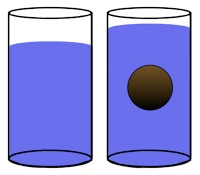Fact 2. When an object is submerged in water, the surrounding water exerts a force (buoyancy force) on the object. This force increases with the depth of the water, so that for any submerged object, there is a net force upwards.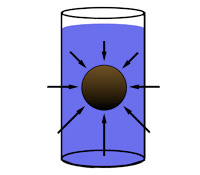Fact 3. Any object is attracted downward due to gravity. This force increases with the mass of the object.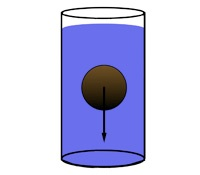There are two primary forces acting on an object placed in water:
1. a gravitational force acts in the downward direction causing the object to sink. The strength of this force depends on the object's mass (weight) -- the more massive an object the stronger the downward gravitational force will be.
2. a buoyancy force acts in all directions, but has a net upwards direction, causing the object to float. The strength of this force depends on how much water the object displaces -- the more water that is displaced the stronger the upward buoyancy force.

If the downward gravitational force is less than the upward buoyancy force then the object floats, otherwise it sinks. That is, if an object weighs less than the amount of water it displaces then it floats otherwise it sinks. A boat floats because it displaces water that weighs more than its own weight.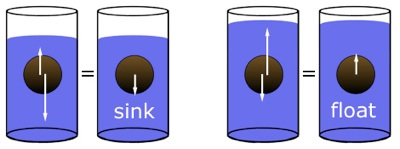WHY DON'T PEOPLE ON THE OTHER SIDE FALL OFF?

Every object in the universe attracts every other object with a force (gravity) that is proportional to the masses of the objects and inversely proportional to the squared distance between objects. Since the earth is so massive it attracts us (no matter where we stand) with a force strong enough to keep us from falling off. Read on for a more detailed explanation.

 Fact 1. Mass is a measure of how much matter is in an object. On earth, mass and weight are effectively the same thing.  Fact 2. The earth's mass  is approximately: 6,000,000,000,000,000,000,000,000 kg or 13,000,000,000,000,000,000,000,000 lb Fact 3. Gravity is an attractive force between any two objects in the universe. The force attracting any two bodies is proportional to the product of their masses and is inversely proportional to the squared distance between them. Below, the gravitational attraction is stronger for the right-most pair of objects because they are more massive and closer together.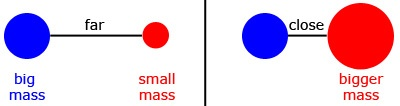The attractive force of gravity acts between the center of two objects. In the case of people standing on the earth's surface, the effect of gravity is to attract us towards the center of the earth. As a result, no matter where you stand on the earth, you don't fall off.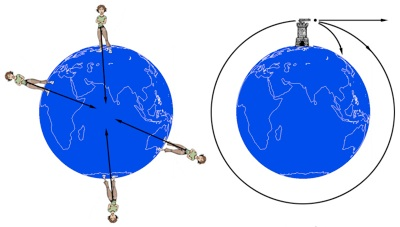Gravity is also the reason why the moon (and satellites) orbit the earth and why we orbit the sun. But then why doesn't the moon crash into the earth? The moon is, in fact, constantly falling towards earth, it is just that it keeps missing. With an initial motion along the path of the orbit, the moon can continually fall towards earth without ever reaching it. This is illustrated on the right in the above diagram, where a cannon is shown firing a cannonball. If the speed of the cannonball is too slow it will crash into the earth. If the speed is too fast, the cannonball will escape the gravitational attraction of the earth. But, if the speed is just right, then the cannon ball will continually fall towards the earth, but never reach it – it will orbit the earth.

 An object's weight is a measure of how much gravity is acting on it. An object weighs less on the moon because the moon's mass, and therefore gravitational attraction, is less. An object's mass, however, is the same no matter where it is.

 A typical plane with people, cargo and fuel has a mass of 70,000 kilograms (kg) (154,322 pounds (lb) or 77.16 tons). If we compare the mass of the earth to the distance between the earth and the sun, then the mass of a plane would be approximately the diameter of an atom.

 Objects will orbit the earth only if they are above earth's atmosphere, where particles in the earth's atmosphere do not slow it down.

WHY DO THINGS LOOK DARKER WHEN THEY ARE WET?

When light strikes an object, some of it penetrates the object, and some of it is reflected and reaches your eye. When an object is wet, more light penetrates the object, so less light is reflected. As a result, less light reaches your eye and so the wet object looks darker. Read on for a more detailed explanation.

 Fact 1. When light moves from air to water, some of the light reflects and some refracts. The reflected light "bounces" off the water, and the refracted light bends at the air/water boundary and passes through the water.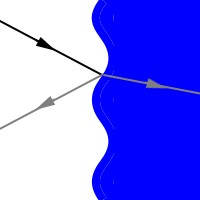Fact 2. When light strikes any object, some of the light is reflected and some is refracted and transmitted through or absorbed by the object. The relative amounts of which depend on the material properties of the object, its index of refraction.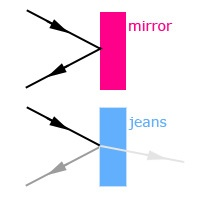Fact 3. When a material gets wet and absorbs water, the material's index of refraction is effectively changed, making it so that more light penetrates and less light is reflected.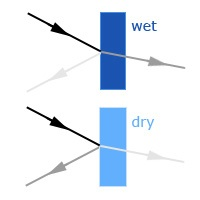The light that is reflected from an object is the light that we perceive. How light or dark an object appears depends on how much light that strikes an object reflects back to our eye. For an object whose material has an index of refraction close to that of air very little light is reflected. For an object whose material has an index of refraction different than air, most of the light that strikes it is reflected.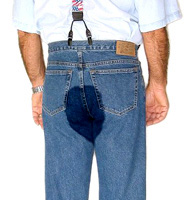When an object gets wet and absorbs water, its index of refraction effectively moves closer to that of air. When light strikes a wet object, therefore, less light is reflected than when it is dry. A pair of wet pants, a wet sidewalk, and a wet beach, therefore, reflects less light, and therefore looks darker. Steel, glass or plastic doesn't look darker when it is wet because it doesn't absorb any water, and therefore the same amount of light is reflected whether it is dry or wet.

WHY IS IT COLDER IN THE WINTER THAN IN THE SUMMER?

The earth's axis of rotation is tilted relative to the earth's path around the sun. As a result we are tilted towards the sun in the summer and away from the sun in the winter. Read on for a more detailed explanation.

 Fact 1. The earth rotates about its axis once every 24 hours. In the morning we are facing towards the sun, and at night we are facing away from the sun.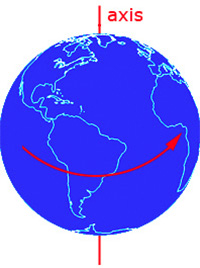Fact 2. The earth rotates around the sun (approximately) every 365 days or 1 year.Fact 3. The axis about which the earth rotates is tilted (by 23.5 degrees) relative to the earth's path around the sun.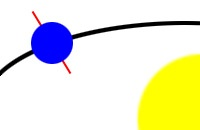Shown below are two diagrams of the earth at the same time of day. On the left it is winter and on the right it is summer (in the northern hemisphere). Notice that the same spot (red circle) in the winter receives much less light than in the summer. As a result, it is colder in the winter than in the summer. (Note: in this diagram, the earth's axis is 33 degrees, instead of 23.5, so as to better illustrate the effect.)